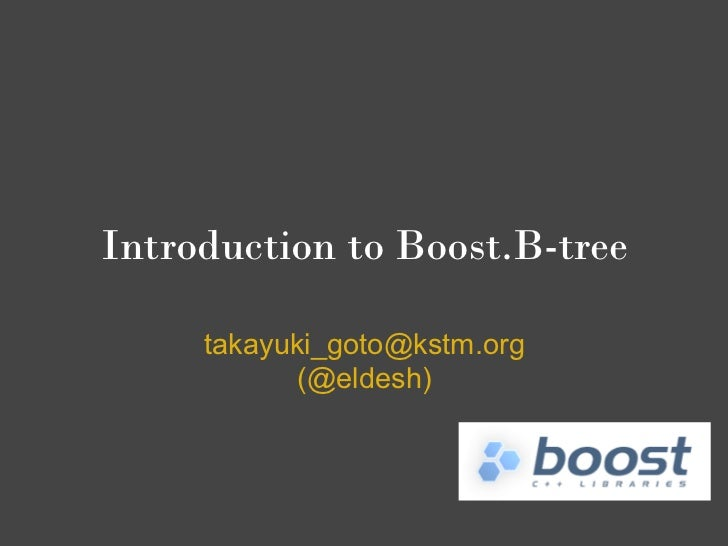Successfully reported this slideshow.Upcoming SlideShare
×

Boost.B-tree introduction

3,081 views

Published on

introduction to Boost.B-tree.
presented on Boost.勉強会#7
http://partake.in/events/a85f6ec3-95c4-40ee-8308-a5746c6277d4

Published in: Technology
• Full Name
Comment goes here.

Are you sure you want to Yes No• Be the first to comment

Boost.B-tree introduction

1. 1. Introduction to Boost.B-tree takayuki_goto@kstm.org (@eldesh)
2. 2. 自己紹介● hatena-id:eldesh, @eldesh● C++/ML er● github ○ Inhert.PEG :: packrat parser library● codeplex ○ msgpack-sml :: SML message-packシリアライザ● 就職活動中なう…。 ○ 拾ってください＞＜
3. 3. 目次● Boost.B-treeとは● B-treeとは ○ 性質 ○ 効率 ○ 採用事例 ● Boost.B-treeの特徴● Boost.B-treeの使い方● Boost.B-treeの実装 、制限
4. 4. B-treeとは● データ構造(C++風に言うとコンテナ)の一種● 古来よりHDD上で(ファイルシステムの実装に)活用される● 近年オンメモリDBとして注目されている[要出典] ○ KyotoCabinetなど● 派生のデータ構造たくさん ○ B+, B*, B#, Bx, UB, Dancing, H
5. 5.
6. 6. B-treeの性質● 平衡多分木の一つ ○ 自動で常にバランスする● 全てのリーフノードはルートから同じ距離になる● ノード数は一定 ● HDD上で性能が出る● SSDでも速い(らしい)
7. 7. B-treeの効率● 他のコンテナとの比較 コンテナ イテレータ メモリ効率 検索 挿入 削除 B-tree bi-directional N*1.25 O(logmN) O(logmN) O(logmN) (最悪N*2) RedBlack-tree bi-directional N*3(or 2) O(log2N) O(log2N) O(log2N) (std::mapなど) SkipList (bi- N*2(？) O(log2N) O(log2N) O(log2N) directional)
8. 8. B-tree採用事例● NTFS(B+-tree)● ext3,4(hashed B-tree)● HFS(B-tree)● Reiser4(Dancing B*-tree)● XFS(B+-Tree)● MySQL(B-tree)● SQLite(B-tree)● BerkeleyDB(B+-tree)● CouchDB(B-tree) ※単に B-tree と言った場合、派生アルゴリズムを含む "B-tree系" の総称として用いることが多いと思われる
9. 9. B-treeとは…！Beman Dawes「B-treeは実質的に、ファイルシステム、リレーショナルデータベース検索機構、NoSQL構造化データシステム、ディスクに保存するインデックス全てにインデックスデータ構造を提供するっ！」 ※強調は訳者による
10. 10. Beman Dawes →
11. 11. Boost.B-tree
12. 12. Boost.B-treeの特徴● std::set, std::mapライクなコンテナ● B+-treeで実装されている● 自動でファイルに同期● ライブラリレベルでのキャッシュ管理● preloadによる高速化オプション● packによるメモリ効率最適化オプション● 格納出来る値に制限がある● 実はBoostに入ってない :p
13. 13. B+-tree
14. 14. インストールgit clone https://github.com/Beman/Boost-Btree.git xbtree(注) Boost全部チェックアウトしますsvn export --force http://svn.boost.org/svn/boost/trunk xbtreecd xbtree./bootstrap.shcd libs/btree/test../../../bjam
15. 15. 動作確認&ベンチマーク\$ cd xbtree/libs/btree/test\$ bjam\$ cd ../tools\$ bjam\$ bin/bt_time 10000 -stl -p4096 -c1000
16. 16. ライブラリファイル構成boost/btreedetail/support/dynamic_size.hpp <- 可変サイズKey,Valueメタ関数header.hpp <- Btreeのヘッダクラスmap.hpp <- btree_map, btree_multimapset.hpp <- btree_set, btree_multiset
17. 17. example/btree_setusing namespace boost::btree;typedef btree_set<int> set_type;set_type s("set.btr", flags::truncate);s.insert(0);s.insert(1);s.insert(2);for (set_type::iterator it=s.begin(); it!=s.end(); ++it) cout << it << n;// output// 0// 1// 2
18. 18. example/btree_maptypedef btree_map<int, long> map_type;map_type m("bt_map.btr", flags::truncate);m.emplace(2, -2); // m.insert(make_pair(2, -2))m.emplace(3, -3);m.emplace(1, -1);for (map_type::iterator it=m.begin(); it!=m.end(); ++it) cout << it->key() << " --> " << it->mapped_value() << n;// 2 --> -2// 3 --> -3// 1 --> -1
19. 19. example/btree_multisettypedef boost::btree::btree_multiset<int>set_type;set_type s("set.btr", flags::truncate);s.insert(1);s.insert(2); s.insert(2); s.insert(2);s.insert(3);set_type::const_iterator_range rng=s.equal_range(2);for (set_type::const_iterator it=rng.first; it!=rng.second; ++it) cout << *it << n;// output:// 2// 2// 2
20. 20. example/btree_multimaptypedef btree_multiset<strbuf,strbuf> map_type;map_type m("map.btr", flags::truncate);m.emplace("eat","comer0");m.emplace("eat","comer1");m.emplace("eat","comer2");m.emplace("drink", "beber");map_type::const_iterator_range rng=m.equal_range("eat");for (map_type::iterator it=rng.first; it!=rng.second; ++it) cout << it->key() <<"-->"<< it->mapped_value()<< n;// output:// eat -> comer0// eat -> comer1// eat -> comer2
21. 21. 標準連想コンテナとの違い● テンプレートパラメータ ○ エンディアンを指定する● Key とTへのより厳しい制約● イテレータの無効化規則は、通常の古い連想コンテナより順序 無し連想コンテナにより近い● [multi]map value_type は std::pair<> ではなく、 map_value<> である● iterator と const_iterator は同じ型であり、それは不 変なイテレータである● いくつかのメンバ関数が削除され、いくつかが追加された
22. 22. Key, Tへの制約btree_map< class Key, class T <- コレ , class Traits=default_endian_traits , class Comp=btree::less<T> > ● static_assert(std::is_trivially_copyable<T>::value==true,"") ○ memcpyします ● ポインタではないこと を要求する。
23. 23. Key, Valueのバイナリ表現template<class T1, class T2>class map_value { // btree_map<>::value_type T1 const& key() const { return *reinterpret_cast<const T1*>(this); } T2 const& mapped_value() const { return *reinterpret_cast<const T2*>( reinterpret_cast<const char*>(this) + dynamic_size(key())); }};// キャストでアクセスするので// std::pair<>は使えない
24. 24. マップされた値の更新 ● iterator と const_iterator は同じ型であり、 不変なイテレータである btree_map::iterator it=m.find(2); it->mapped_value() = "hoge"; // error ● 値の更新は専用メソッドを使うiteratorbtree_map<K,V>::update(iterator, V const&);
25. 25. example/updatetypedef btree::btree_map<int, long> map_type;map_type m("bt_map.btr", btree::flags::truncate);m.emplace(2, -2);m.emplace(3, -3); m.emplace(1, -1);map_type::iterator it=bt_map.find(2);//じつはconst_iteratorでも…cout << it->mapped_value() << "n";m.update(it, 20L);cout << it->mapped_value() << "n";// -2// 20
26. 26. Boost.B-treeでは出来ないこと● std::stringは使えない ○ trivially_copyableをkey,valueに要求する ○ 文字列を使うためのwrapperはある● コンテナに値を追加すると勝手にファイルに書き出される● シリアライザでは無い ○ Boost.Serializationの代替ではない ○ 複雑なデータ型はB-treeの上に構築する
27. 27. 追加された関数(の一部) void open(const path& p, flags::bitmask flgs = flags::read_only, size_t node_sz = default_node_size) // node_sz ignored if existing file void flush(); void close(); bool is_open() const; size_t node_size() const; size_t max_cache_size() const; void max_cache_size(size_t m); void dump_dot(std::ostream &) const;
28. 28.  digraph btree {rankdir=LR;fontname=Courier;node [shape = record,margin=.1,width=.1,height=.1,fontname=Courier,style="filled"];node1[label = "<f0> 0|1|2|3|4|5|6|7|8|9",fillcolor="palegreen"];}
29. 29.
30. 30. クラス階層
31. 31. ページキャッシュbuffer_manager // ファイルとバッファの管理 ● intrusive::set<buffer> // と ● intrusive::list<buffer> // で管理
32. 32. Feedback welcome!● まだまだ開発途中● 名前もシグネチャも変わるかも知れない● ドキュメントがぜんぜん無い● 継続的に開発していくよ● フィードバック歓迎！
33. 33. ReferencesOrganization and Maintenance of Large Ordered Indexes,Bayer and McCreight, 1972https://github.com/Beman/Boost-BtreeProposed Boost.B-tree Library(ja)